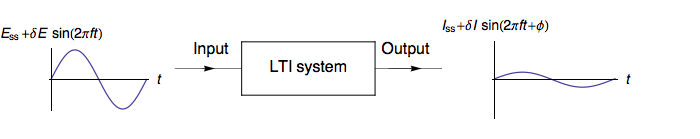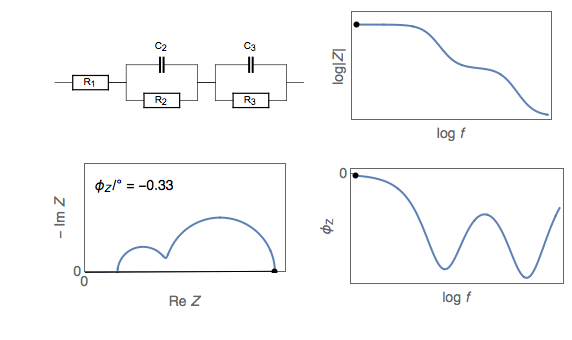```Only 10\$ for interpretation of your EIS spectrum
Payment Upon Completion

Electrochemical Impedance Spectroscopy (EIS) is a powerful tool enabling the study of processes that occur at the interface of an electrode.

In EIS a periodic signal is applied in current or voltage at several frequencies. The periodic signal is traditionally built with a sinus.The transfer function H of the system is defined as:

H(s)=L[Output(t)]L[Input(t)]H(s)=ℒ[Output(t)]ℒ[Input(t)] (1)

ℒ  being the Laplace transform

Measurements as a function of the frequency of the perturbation give an impedance, Z,  (or admittance) diagram. The impedance is given in Ohm as it is the ratio of the voltage vs. the current and is a complex number.

To be valid, the system under study has to be:

• Linear: The response (output) of the cell has to be directly proportional to the input. The small perturbation of the electrode state has the advantage that the solutions of relevant mathematical equations used are transformed in linear forms.
• Time invariant: The state of the cell must not change during the measurements.
• Causal: The output has to be correlated directly with the input.

The modulus Z and phase Phi are the parameters of interest, so the impedance data can be plotted in Bode plot (Z and Phi vs frequency), but in electrochemistry, the most common plot is the Nyquist plot -Im(Z) vs Re(Z).As the periodical perturbation is performed at several frequencies, EIS is capable of characterizing processes that have different time constants i.e. fast process at high frequency (> 10 kHz) and low process such as diffusion at low frequencies (<100 mHz).

Consider Ohm’s law, which describes the relationship of voltage to a direct current passing through a resistor:

E=IRE=IR

Impedance is, very simply, extends the concept of resistance to an alternating current circuit, and generally represented as ZZ. So you can think of it, simply, like this:

E=IZZE=IZZ

We’ll come back to this in a moment. For now, it should be clear that a measurement of impedance, therefore, can be made by made simply by applying an oscillating voltage, and measuring the (oscillating) current response. We can write down an equation for the oscillating voltage we apply like so:

E(t)=|E|sin(ωt)E(t)=|E|sin⁡(ωt)

where |E||E| is the amplitude of the voltage signal, and ω=2πfω=2πf (the angular frequency). The response will be a current with an amplitude |I||I|, which is also shifted in phase from the applied signal:

I(t)=|I|sin(ωt+θ)I(t)=|I|sin⁡(ωt+θ)

The current is shifted in phase because of reactance (e.g., a capacitance or inductance) in addition to the resistance (which changes the amplitude). The impedance can therefore be expressed like this:

ZZ=E(t)I(t)=|E|sin(ωt)|I|sin(ωt+θ)=|Z|sin(ωt)sin(ωt+θ)ZZ=E(t)I(t)=|E|sin⁡(ωt)|I|sin⁡(ωt+θ)=|Z|sin⁡(ωt)sin⁡(ωt+θ)

Have a look at the animation below. The ‘current’, I, is 72° out of phase with the ‘voltage’. The graph on the right is known as a Lissajous curve, showing the relationship between I and E. In the past, impedance spectroscopy was done by obtaining these curves on an oscilloscope and analysing them. Thankfully, it’s all a bit easier nowadays.

## Complex representation

Ok, complex maths time. Without going into too much detail, via Euler’s formula:

ejx=cos(x)+jsin(x)ejx=cos⁡(x)+jsin⁡(x)

we can re-write all of the above using complex numbers:

ZZ=|Z|ejθ=|E|ejωt|I|ejωt+θZZ=|Z|ejθ=|E|ejωt|I|ejωt+θ

or simply:

EE=IZZ=I|Z|ejθEE=IZZ=I|Z|ejθ

Note that jj is the imaginary unit, i.e., j=√−1j=−1, which we use instead of ii to avoid confusion with the symbol for electrical current. You can see from the above equation that the ratio of an oscillating voltage to an oscillating current is the impedance, which has a magnitude |Z||Z| and a phase angle θθ. You can think of this as a polar coordinate representation. More commonly for impedance spectroscopy, however, we generally use the Cartesian complex plane representation, dividing the complex impedance into the real and imaginary parts:

ZZ=Z′+jZ′′ZZ=Z′+jZ″

Z′Z′ and Z′′Z″ are the resistive and reactive parts of the impedance respectively. You’ll see this more clearly on the page about the impedance of simple RC circuits.

We can represent any ZZZZ on an Argand diagram, as in the graph below. This is the basis for the Nyquist plot, which is the plot of the real and imaginary parts of the impedance that you’ll come across most often. An impedance measurement for a single frequency is a single point on a Nyquist plot. An impedance spectrum is therefore a series of points, where each point is a different frequency.

These plots are visually useful, because the characteristic shapes that can appear in the plots as you’ll see later can give you a rough idea of what you’re looking at. The downside, though, is that you can’t know what the frequency associated with a particular point is from looking at the Nyquist plot alone, and so the plot doesn’t contain all the information you need. This is why the alternative Bode plot – plots of logZ′log⁡Z′ and logZ′′log⁡Z″ vs logflog⁡f, or log|Z|log⁡|Z| and θθ vs logflog⁡f – are still important.

## Nyquist plot

I’ll finish up this page by briefly introducing a typical Nyquist representation of an impedance spectrum itself. The plot below is data I acquired from a Li-ion test battery, and fitted to a model myself. The frequency range the points represents is between 100 kHz and 100 mHz. This is fairly typical for most systems, although depending on what you want to measure you might go up to 1 MHz or more, or as low as 1 mHz. So how do you make sense of this plot? Well, there are three things I’ll note for now.

First, the impedance is always lowest (i.e., smallest values of |Z||Z| at the highest frequency, so you can see that the frequency decreases if we follow the curve from the points near the origin to the points in the top-right corner. Secondly, you’ll note (as in the Argand diagram above) that the values of Z′′Z″ are negative (plotted as −Z′′−Z″). This will become clearer later, but by convention capacitance is a negative reactance, so impedance spectra will in most cases only have positive Z′Z′ values and negative Z′′Z″ values.

Lastly, you’ll note the shape of the spectrum, particularly the semi-circle part. The shapes you see in the Nyquist plots can be characteristic of certain elements or combinations of elements, so they are (often, but not always) visually useful for quickly understanding something about the system you’re measuring. Because of this I was able to take this relatively good quality data, think of a reasonable model, guess a few of the parameters and then fit the entire spectrum relatively quickly. In the following pages you’ll read about the experimental technique I used to get this data as well as the elements of the model I’ve fitted the data to, and hopefully you’ll be able to see how it all fits together.

Orthonormal scales should be used for Nyquist impedance plots. The length from 0 to 1 along the imaginary axis should be equal to the length from 0 to 1 along the real axis. Otherwise, semicircle graphs are not semicircles (Fig. 1) and it becomes difficult to measure angles (Fig. 2). We present here two examples: the impedance for a Tafelian redox system (Fig. 1) and the Randles circuit with Warburg impedance (Fig. 2). Orthonormal and non-orthonormal plots are compared: non-orthonormal scales are indicated by a sad face and the orthonormal scale is indicated by a happy face. More details in the corresponding Mathematica Demonstration .

EIS accuracy contour plots must be used to interpret errors made during EIS measurements and identify the best frequencies possible to be used for a given impedance range. The aim of Fig. 1 is to demonstrate how to read and understand EIS accuracy contour plots provided with each potentiostat .

Fig 1: Superimposition of EIS accuracy contour plot and modulus
Bode diagram of R1+R2/C2  electrical circuit (left) and Nyquist diagram
of the same circuit (right).

The different colored areas show different ranges of impedances that can be measured at various frequencies within a specified error in magnitude (%) and in phase (°).

The accuracy contour plot is an image of the capability of the instrument and is only valid for a given set of conditions (input amplitude, temperature, data averaging, etc).